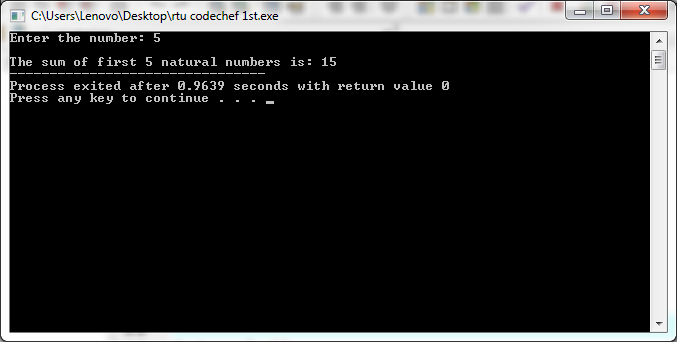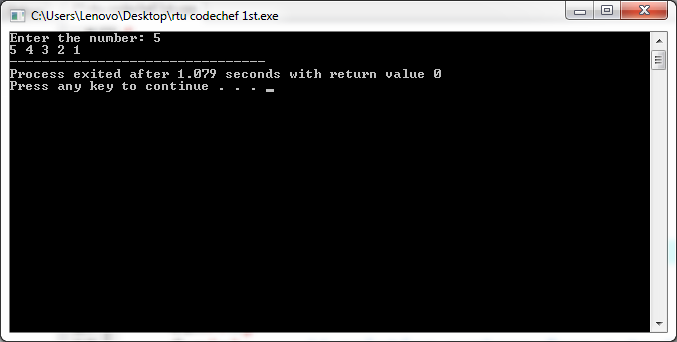# Learning about C++ Recursion and its application

By NIIT Editorial

Published on 16/08/2021

7 minutes

Recursion happens when, directly or indirectly, the function calls itself along with the corresponding function known as the recursive function. This process is usually taken into use when complex iterative tasks need to be divided into sub-tasks. The process of recursion possesses multiple iterative cells for the same function. For terminating a recursion, having a base case is of utmost importance.

Simply stated, if a complex mathematical computation task needs to be solved, it can be done with the help of Recursion through the division of tasks into sub-tasks. This whole phenomenon of solving complex tasks is known as Divide and Conquer. Even for the programmers, it is a boon. They are enabled to split the complex problem into multiple sub-tasks. These are then solved individually for reaching the final solution.

Even though the tasks can also be solved through the iterative method, Recursion still remains the most efficient programming method. This is because not much coding is needed to perform similar complex tasks. However, note that Recursion cannot be an overall approach to solve all kinds of problems. It is the most suitable for issues like searching, sorting, DFS of Graph algorithms and Inorder/Postorder/Preorder Tree Traversals. Make sure that the entire process of Recursion is implemented precisely or else it may end up in an infinite loop and will then terminate the function because of not meeting the conditions.

Functioning of Recursion

Precise understanding of the whole Recursion process can be done by analysing a very common example of Recursion. First things first, calculate the factorial of n numbers. Express the factorial of n numbers as a repetitive multiplication series, just like the one shown below:

1)      Factorial of a number = n! = n*(n-1)*(n-2)*(n-3)….1

Example:

Factorial of 3 = 3*2*1 = 6

Factorial of 4 = 4*3*2*1 = 24

Factorial(4) = 24

Return 4*Factorial(3) = 24

Return 3*Factorial(2) = 6

Return 2*Factorial(1) = 2

Return 1

What is Recursive Function?

There is not much difference in the Recursive function as compared to the normal ones. As it is just a function that calls itself both directly or indirectly. A recursive function has the ability to be repetitive and compute the final return output. A recursive function is commonly popular amongst programmers as they make the computer programming process smoother. It also enables the programmers to effectively do minimal coding while writing efficient programs. Let’s have a detailed look at the characteristics of recursive functions:

Features of Recursive Functions

-       If it’s a recursive function, it will always call itself.

-       The stack overheads of a recursive program slow itself.

-       A recursive function must possess recursive expressions and terminating conditions/base case.

Let’s have a look at the basic syntax of recursion:

void fun()

{

……                           // some statements

fun() ;

……

}

int main()

{

…..

fun() ;

…..

return 0;

}

Recursion – types and examples

Below-mentioned are the two types on which the recursive functions are based, have a look:

Direct Recursion: When a recursive function directly calls itself then that is known as a direct recursive function and the recursion that occurs is known as direct recursion.

void fun()

{

……

fun() ;    // function calling itself directly

…..

}

Indirect Recursion:- When a recursive function indirectly calls itself then that is known as an indirect recursive function and the recursion that occurs is known as indirect recursion.

void fun1()

{

…..

fun2() ;       // fun1() calling fun2()

..…

}

void fun2()

{

…..

fun1() ;      // fun2() calling fun1()

…..

}

Memory Allocation in Recursion

Memory allocation for the recursive function is just similar to the other functions. A recursive function calls itself and allocates a single memory block in stock. The required memory is held by the memory block in order to make the execution successful and also to stack up the automatic, local and temporary variables. The separate stack is pushed in the same manner whenever each recursive function is called. If it is called 5 times, then 5 stacks of frames would be corresponding to each of the recursive calls. Once the existing recursive call is ended, the new stack of frames gets discarded and the function starts to return its value corresponding to the function in the previous stack frame. This stack frame then pops out in the same way it was pushed. The memory then gets de-allocated. This process is repeated until the final call is reached. Finally, at the end of the recursion call, the final result value is returned to the original call and the stack is destroyed, freeing up the memory. If in case the recursion is not able to reach the base case, then again, the stack will quickly be exhausted which will lead to a stack overflow crash.

While there are advantages of recursion like – requiring few variables to make a clean program and shortens the complex/nested code; there are quite a few disadvantages too, such as:

-       Difficulty in debugging the recursive function

-       Difficulty in understanding the straight logic of a recursive function

-       An infinite loop can be caused or even unexpected results can occur if the code is not written precisely.

-       A recursive program requires a large amount of memory space as compared to the iterative program.

-       These recursive programs are comparatively slower than the non-recursive ones.

Example: Sum of the first N natural numbers using recursion.

// Sum of first n natural numbers using recursion

#include<iostream>

using namespace std;

int fun(int n)              // function returning the sum of natural numbers

{

if(n==1)

return 1;

return n+fun(n-1);

}

int main()

{

int n;

cout<<"Enter the number: ";

cin>>n;

cout<<"\nThe sum of first "<<n<<" natural numbers is: ";

cout<<fun(n);

return 0;

}

Output:Here n = 5, so the program execution goes as follows:

First fun(5) will be called, then it it see the condition that whether n is equals to 1 or not. Here it is not so it will call fun(4) and so on. When n becomes 1 it will return 1.

Fun(5) = 5+fun(4)    // will return 5 + 10 = 15

Fun(4) = 4+fun(3)    // will return 4 + 6 = 10

Fun(3) = 3+fun(2)   // will return 3 + 3 = 6

Fun(2) = 2+fun(1)   // will return 2 + 1 = 3

Fun(1) will return 1

Understanding tail recursion

When a recursive function has its recursive call as the last statement to be executed the recursion form is called tail recursion and the function is tail-recursive. The tail-recursive functions are generally considered more effective than the non-tail recursive functions as the compiler can optimize them easily. This is because the recursive call is the last statement and therefore you do not need to track the current state of the function in the given stack frame. You can learn more about recursive function in the courses  PGP in Full-Stack Product Engineering and PGP in Full Stack Software Engineering that are offered by NIIT.

#include<iostream>

using namespace std;

void fun(int n)

{

if(n==0)

return;

cout<<n<<" ";

fun(n-1);                       // recursive call to be executed

}

int main()

{

int n;

cout<<"Enter the number: ";

cin>>n;

fun(n);                  // printing numbers from n to 1

return 0;

}

Output:### PGP in Full Stack Product Engineering

Become an Industry-Ready StackRoute Certified Full Stack Product Engineer who can take up Product Development and Digital Transformation projects. Job Assured Program* with a minimum CTC of ₹7LPA*

Job Assured Program*

Easy Financing Options